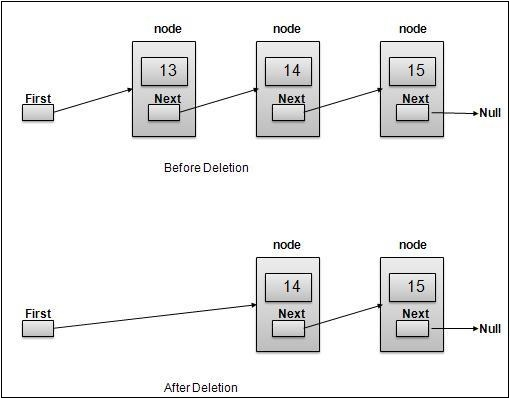# Remove elements from a linked list using Javascript

Removing an element is very easy in a linked list. We just need to get rid of the node we want to remove, ie, lose its reference. There are 3 cases we need to consider −

• Removing an element from head: In this case, we can simply assign head = head.next. This way we'll lose the reference of the first element. And out head will start pointing to the second element.
•  Removing an element from the tail: In this case, we can simply assign the node.next of second last node to be null and we'll get rid of the last element from the list.
•  Removing an element from in between: This is more tricky. In this case, we'll have to make the node before the node we want to remove, to directly point to the node after the node we want to remove. So, prevNode.next = node.next will do this for us.

Now let's see an illustration of this −Now let's have a look at how we'll implement this −

## Example

remove(data, position = 0) {
if (this.length === 0) {
return;
}
this.length--;
// Condition 1
if (position <= 0) {
}
// Condition 2
else if (position >= this.length - 1) {
while (currNode.next.next != null) {
currNode = currNode.next;
}
currNode.next = null;
}
// Condition 3
else {
let iter = 0;
while (iter < position) {
currNode = currNode.next; iter++;
}
currNode.next = currNode.next.next;
}
}

You can test this using −

## Example

let list = new LinkedList();
list.insert(10);
list.insert(20);
list.insert(30);
list.remove(1);
list.display();
list.insert(15, 2);
list.remove();
list.display();

## output

This will give the output −

20 ->
30 ->
30 ->
15 ->